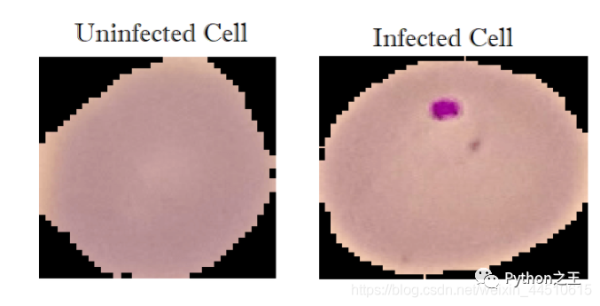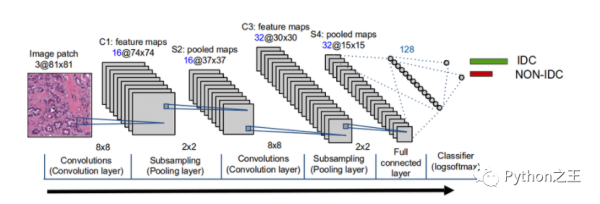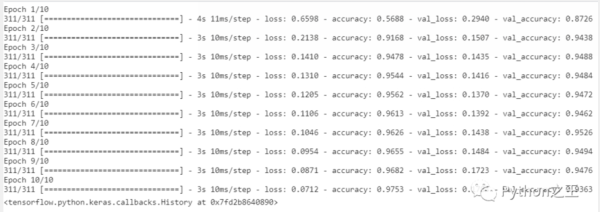# 教你使用TensorFlow2判断细胞图像是否感染import cv2 import tensorflow as tf from tensorflow.keras.models import Sequential  from tensorflow.keras.layers import Dense, Conv2D, MaxPool2D, Flatten, Activation from sklearn.model_selection import train_test_split import numpy as np import matplotlib.pyplot as plt import glob import os

img_dir="../input/cell-images-for-detecting-malaria/cell_images"   img_size=70 def load_img_data(path):     # 打乱数据     image_files = glob.glob(os.path.join(path, "Parasitized/*.png")) +                    glob.glob(os.path.join(path, "Uninfected/*.png"))     X, y = [], []     for image_file in image_files:         # 命名标签  0 for uninfected and 1 for infected         label = 0 if "Uninfected" in image_file else 1         # load the image in gray scale 变成灰度图片         img_arr = cv2.imread(image_file, cv2.IMREAD_GRAYSCALE)         # resize the image to (70x70)  调整图片大小         img_resized = cv2.resize(img_arr, (img_size, img_size))         X.append(img_resized)         y.append(label)     return X, y X, y = load_img_data(img_dir)

print(X.shape)

X的shape为(27558, 70, 70, 1)，27558表示图片的数据，70*70表示图片的长和宽像素。X, y = load_img_data(img_dir) # reshape to (n_samples, 70, 70, 1) (to fit the NN) X = np.array(X).reshape(-1, img_size, img_size, 1) #从[0，255]到[0，1]缩放像素 帮助神经网络更快地训练 X = X / 255  # shuffle & split the dataset X_train, X_test, y_train, y_test = train_test_split(X, y, test_size=0.1, stratify=y) print("Total training samples:", X_train.shape) print("Total validation samples:", X_test.shape)# train the model with 10 epochs, 64 batch size model.fit(X_train, np.array(y_train), batch_size=64, epochs=10, validation_split=0.2)loss, accuracy = model.evaluate(X_test, np.array(y_test), verbose=0) print(f"Testing on {len(X_test)} images, the results are  Accuracy: {accuracy} | Loss: {loss}")

Testing on 2756 images, the results are Accuracy: 0.9404934644699097 | Loss: 0.1666732281446457

model.save("model.h5")

## 官方微博/微信↑扫描二维码

1.用手机扫左侧二维码；

2.在添加朋友里，搜索关注TechWeb。